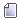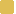Author Topic: Error max2008 function kill and block  (Read 2027 times)

pme

• Full Member
•• Posts: 13Error max2008 function kill and block
« on: February 01, 2008, 04:36:26 PM »
Hello,

We have on the other hand many problems to calculate of max2008.
If we calculate, for the first time on a knot, a file max2008 in render max, the window of render opens on the knot of calculate (black window of render + window of promotion of render) and closes at once without calculating anything, it does not work!
On the other hand, if we calculate a render max2008 there mentalray (on the same knot), the window of render opens on the knot of calculate (black window of render + window of promotion of render), calculates it work.

Since we calculated first time mentalray, we can calculate a render max2008 with max engine (on the same knot), it works !!!! By respecting this method???

I do not understand why it is absolutely necessary to calculate there maxmentalray to make a sort of initialization of the knot of calculate before being able to calculate as we want on the same knot?If we stop a render by the function " kill ", the black window of render + window of promotion of the render remains motionless. It is necessary to close these windows directly on the knot of calculate! And Windows indicates that the program max does not answer.

Can give me myself explanations on these problems.

We also use maya. No problem for calculate them, except for the posting
Calculated images (tga) in "output". We have the following error message: " cannot parse dated for test.tga image "On max, the posting works well (tga).